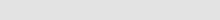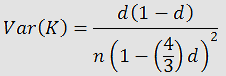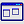Insilicase
deus ex computaChromosome lengthsConsensus site finderDegenerate motif finderExon finderFusion protein (RE)Fusion protein (PCR)Genetic distanceHeterozygous InDelsInvert sequenceList comparisonMicrosatellite peaksMismatch TmMutagenesis (silent)Mutation severityORF annotationPhred score calculationProtein cleavage fragmentsRandom sequenceResidue codes etcRestriction sitesRFLP enzyme pickerStats decision treeAny ideas?Contact me# Jukes-Cantor genetic distances

This page calculates the genetic distance and its variance between two sequences using the Jukes-Cantor model. Rather than create the alignment, it is assumed that you have done this and already know the number of changes and the length of the sequences.

 Number of changes Length of sequences Genetic distance: Variance of genetic distance:

The genetic distance between two sequences is the sum of the substitutions that have accumulated between them since they diverged from their common ancestor. While it is possible to count the differences between the two sequences, this would under estimate the true divergence since some positions may have undergone multiple changes. To overcome this problem Charles Cantor and Thomas Jukes developed a probabilistic model and derived the following formula to calculate genetic distances:where K is the genetic distance and d is the number of observed substitutions divided by the sequence length. They also found the variance of K could be found by:A Windows program that duplicates this page can be downloaded here.